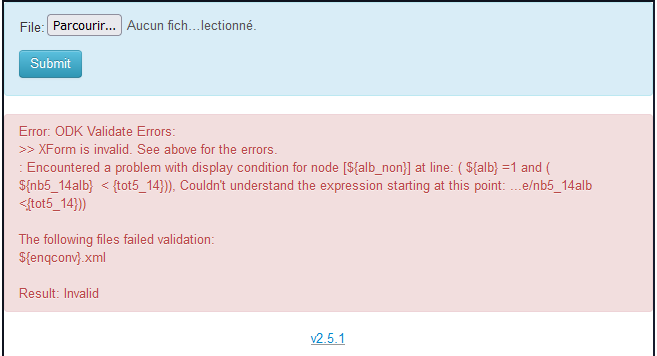# Building Form via XLSForm

Hello every one,
I am building a form via XLSForm. How can I express these 2 conditions bellow in relevant colon:
\${a}=n and \${b} < \${c}

\${a}=n and \${b} < \${c} or \${a}=n and \${d} < \${e}

I noticed that there is error in the way I write them so validate errors appear.

(\${alb}=1 and (\${nb5_14alb} < {tot5_14}))

((\${iver}=1 and (\${nb5_14iver} < {tot5_14})) or (\${iver}=1 and (\${nb15_iver} < {tot15_plus}))

((\${praz}=1 and \${nb5_14prz} < {tot5_14}) or (\${praz}=1 and \${nb15_prz} < {tot15_plus}))You have \$ missing before some {…} references. For example syntax must be … < \${tot5_14}

1 Like

You’re right. I have already correct it But the problem is not solve for the moment.

@mtn2020_2030, maybe it would be easy for the community if you extract the xlsform that holds this expression. Note, please do not share the entire xlsform. You could only share the portion that has this issue.

1 Like

ComHelp.xlsx (13,4 Ko)

Hi @mtn2020_2030, I got this message (after I added a final `end group` to your form)

So you just had an unclosed bracket on line 22:

line relevant
22 old ((\${iver}=1 and (\${nb5_14iver} < \${tot5_14})) or (\${iver}=1 and (\${nb15_iver} < \${tot15_plus}))
22 fix (\${iver}=1 and (\${nb5_14iver} < \${tot5_14})) or (\${iver}=1 and (\${nb15_iver} < \${tot15_plus}))
3 Likes

Many thanks. It’s OK2 Likes